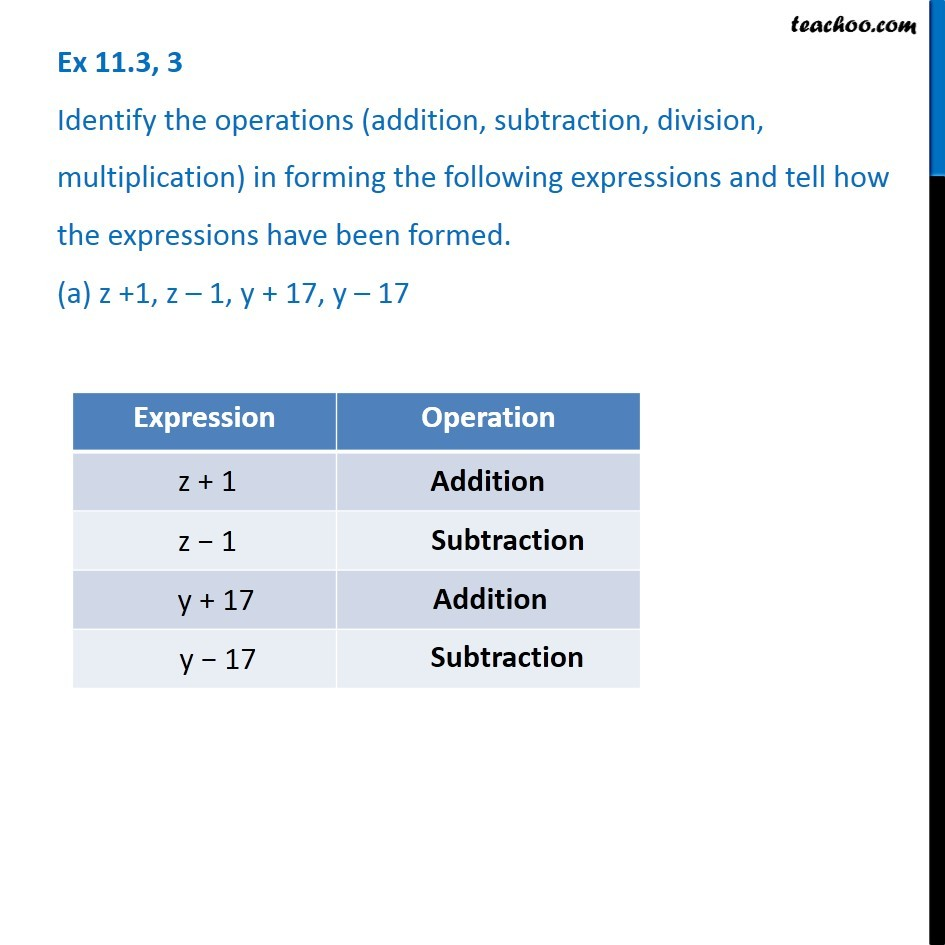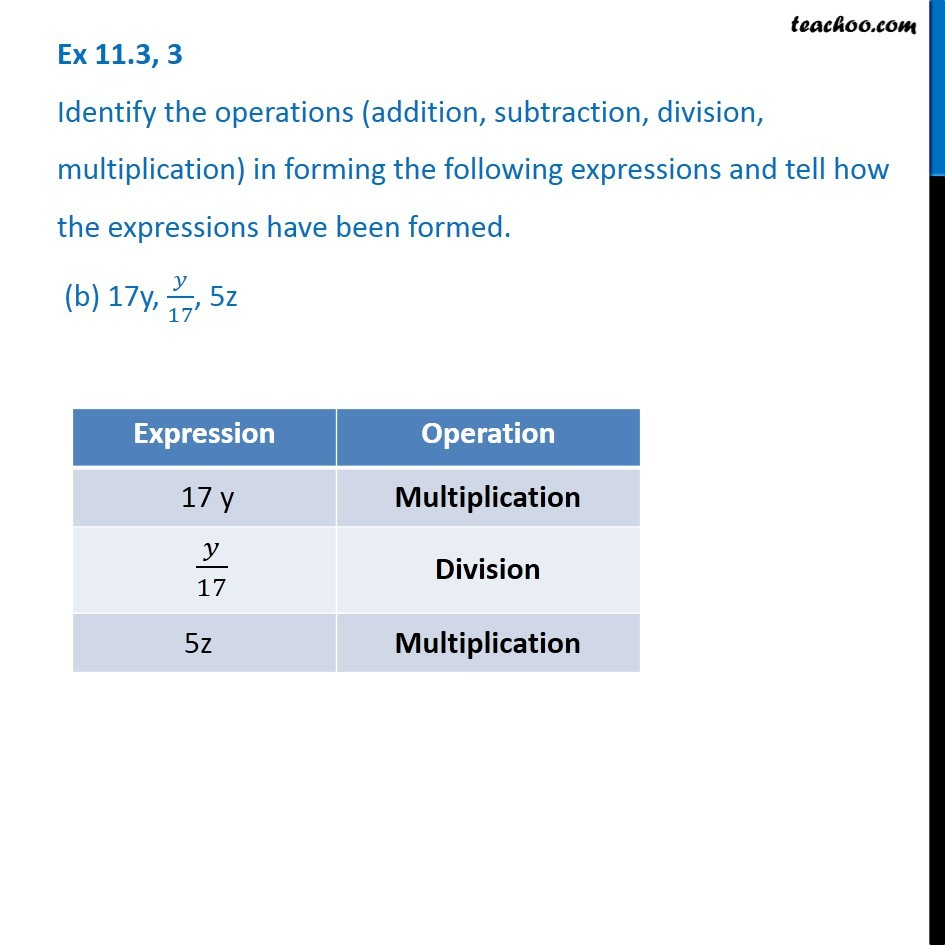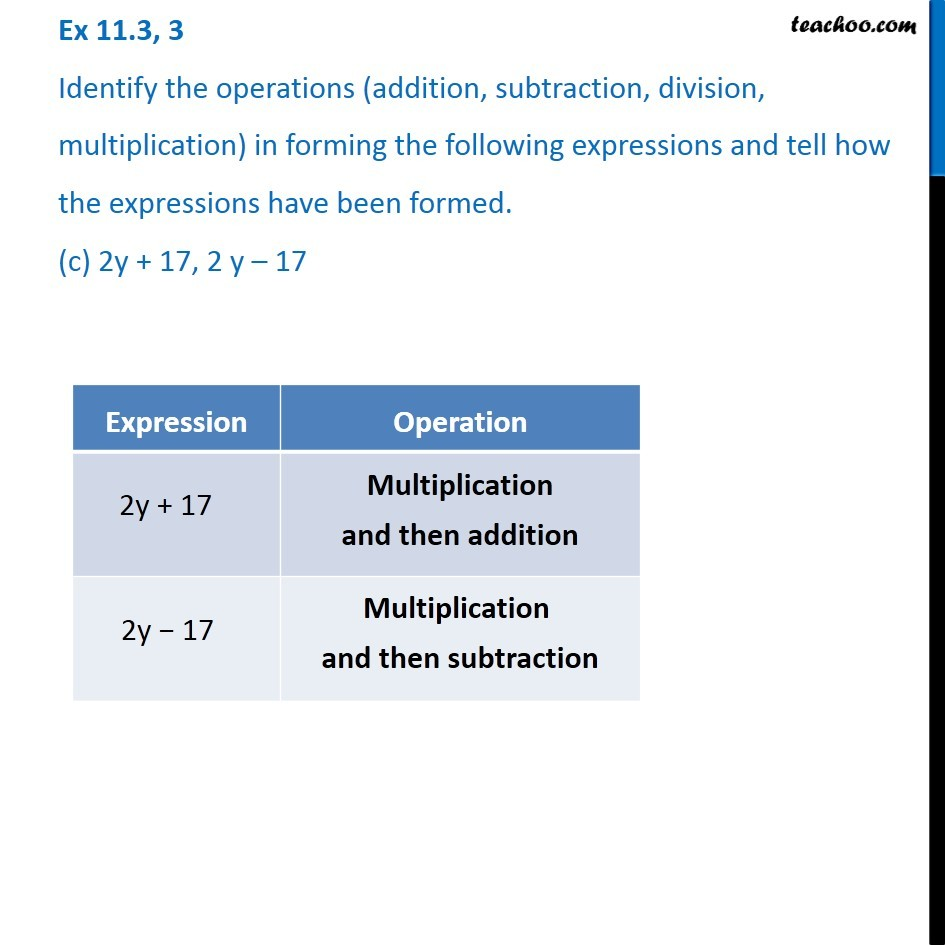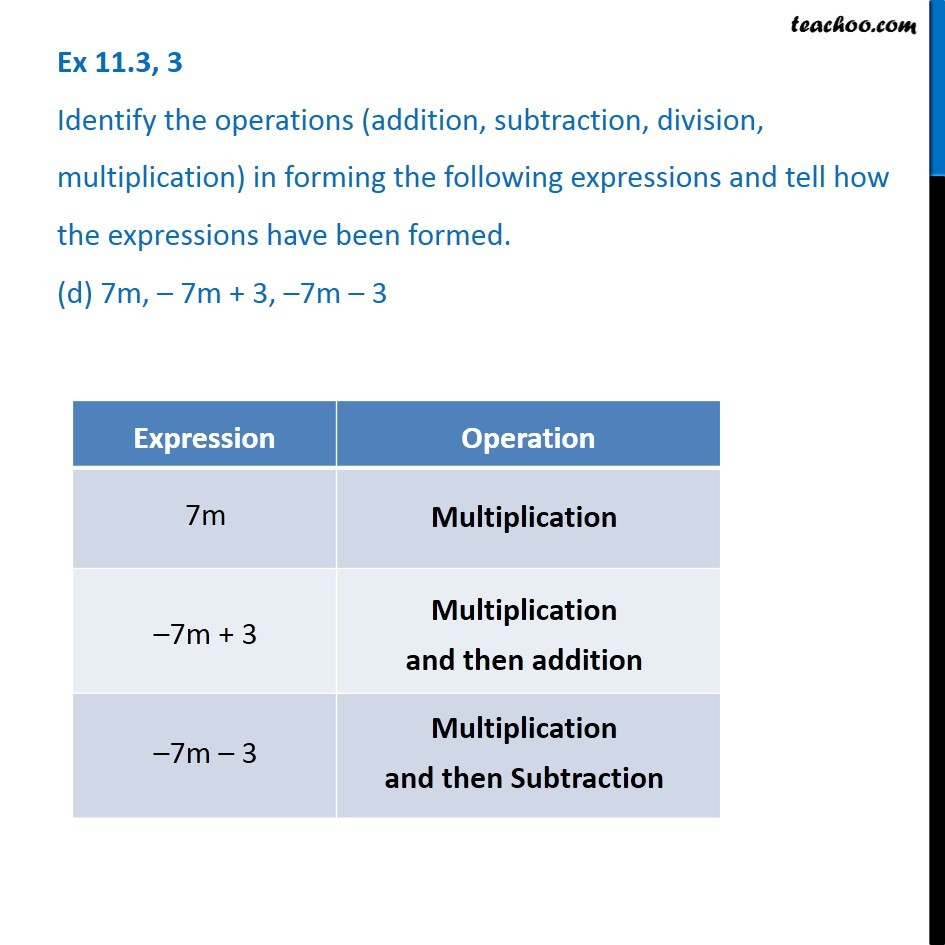Expression with variables

Chapter 11 Class 6 Algebra
Concept wiseIntroducing your new favourite teacher - Teachoo Black, at only ₹83 per month

### Transcript

Ex 11.3, 3 Identify the operations (addition, subtraction, division, multiplication) in forming the following expressions and tell how the expressions have been formed. (a) z +1, z – 1, y + 17, y – 17 Ex 11.3, 3 Identify the operations (addition, subtraction, division, multiplication) in forming the following expressions and tell how the expressions have been formed. (b) 17y, 𝑦/17, 5z Ex 11.3, 3 Identify the operations (addition, subtraction, division, multiplication) in forming the following expressions and tell how the expressions have been formed. (b) 17y, 𝑦/17, 5z Ex 11.3, 3 Identify the operations (addition, subtraction, division, multiplication) in forming the following expressions and tell how the expressions have been formed. (d) 7m, – 7m + 3, –7m – 3